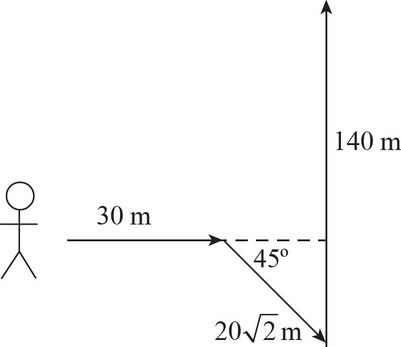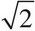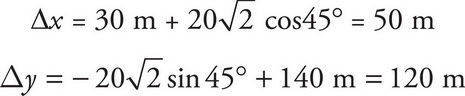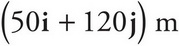# AP Physics 1 Question 419: Answer and Explanation

### Test Information

Question: 419

7. Question below refers to the following scenario:An explorer travels 30 m east, then 20m in a direction 45° south of east, and then 140 m north.

What is the displacement of the explorer?

• A. 130 m
• B. 169 m
• C. 170 m
• D. 215 mThe displacement of the explorer then is the magnitude of the vector: